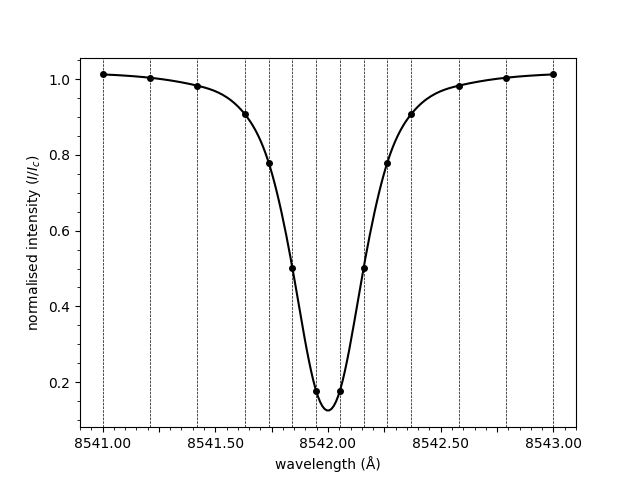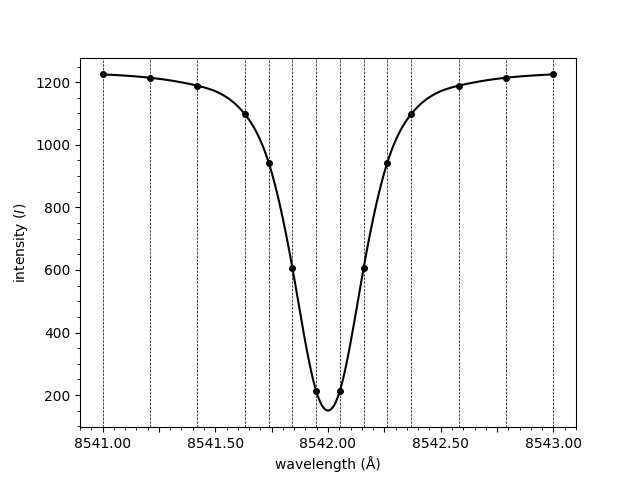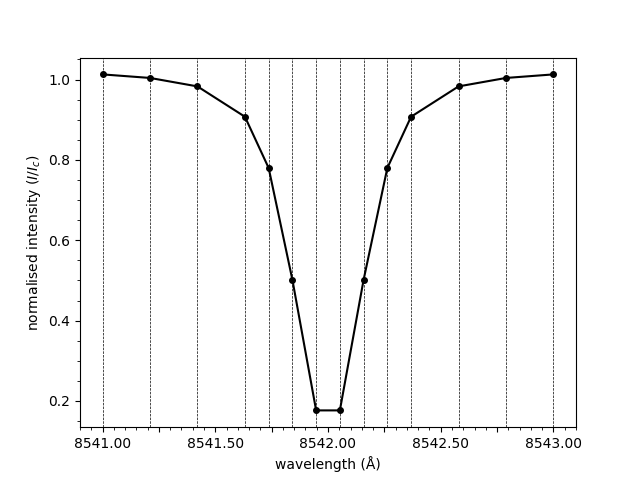# Plot a spectrum

This is an example showing how to plot a spectrum with the `mcalf.visualisation.plot_spectrum()` function.

First we shall create a list of wavelengths, with a variable wavelength spacing. Next, we shall use the Voigt profile to generate spectral intensities at each of the wavelength points. Typically you would provide a spectrum obtained from observations.

```import numpy as np
wavelengths = np.linspace(8541, 8543, 20)
wavelengths = np.delete(wavelengths, np.s_[1:6:2])
wavelengths = np.delete(wavelengths, np.s_[-6::2])

from mcalf.profiles.voigt import voigt
spectrum = voigt(wavelengths, -526, 8542, 0.1, 0.1, 1242)
```

Next, we shall import `mcalf.visualisation.plot_spectrum()`.

```from mcalf.visualisation import plot_spectrum
```

We can now simply plot the spectrum.

```plot_spectrum(wavelengths, spectrum)
``````<Axes: xlabel='wavelength (Å)', ylabel='normalised intensity (\$I/I_c\$)'>
```

Notice how the spectrum above is normalised. The normalisation is applied by dividing through by the mean of the three rightmost points. To plot the raw spectrum,

```plot_spectrum(wavelengths, spectrum, normalised=False)
``````<Axes: xlabel='wavelength (Å)', ylabel='intensity (\$I\$)'>
```

The line connecting the points provided in the `spectrum` array above is smooth. This is due to spline interpolation being applied. Interpolation can be disabled, resulting in a straight line between each of the points.

```plot_spectrum(wavelengths, spectrum, smooth=False)
``````<Axes: xlabel='wavelength (Å)', ylabel='normalised intensity (\$I/I_c\$)'>
```

Total running time of the script: ( 0 minutes 0.845 seconds)

Gallery generated by Sphinx-Gallery